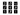← Go BackMay 02, 20205 min read • IN tech

## Preamble

I do not have a lot of experience with Python and the best practices for Python development. I hope to learn more and have been reading about Python development.

#### Aside

Coming from a JavaScript background I find Python, the language itself, amazing, but the package management system, compared to npm, very poor. Pip and virtual environments are quite convoluted.

### Using Matrices for More Efficent Stochastic Gradient Descent

Micheal Nielson intentiollay wrote the code to be slow to show the power of matrices and numpy. The way he wrote it does not utlize the numpy matrix performance, all of which is written to be very fast. To my knowledge numpy is a C binding). The performance gains were very noticeable for me and could be improved much more by trying to use cupy a numpy like interface that utlizes nvidia’s cuda tools to improve performance by off loading matrix operations to the GPU.

Here is an example image of a subset of nodes in the network:Given a mini batch with $m$ number of images:

$\begin{gathered} w = \begin{bmatrix} w_{\textcolor{#a61e4d}{1}\textcolor{#5f3dc4}{1}} & w_{\textcolor{#a61e4d}{1}\textcolor{#2b8a3e}{2}} & w_{\textcolor{#a61e4d}{1}\textcolor{#e67700}{3}} \\ w_{\textcolor{#1864ab}{2}\textcolor{#5f3dc4}{1}} & w_{\textcolor{#1864ab}{2}\textcolor{#2b8a3e}{2}} & w_{\textcolor{#1864ab}{2}\textcolor{#e67700}{3}} \end{bmatrix}\\ a = \begin{bmatrix} \text{Image One} & \text{Image Two} & \dots & \text{Image m} \\ a_{\textcolor{#5f3dc4}{1}1} & a_{\textcolor{#5f3dc4}{1}2} && a_{\textcolor{#5f3dc4}{1}m}\\ a_{\textcolor{#2b8a3e}{2}1} & a_{\textcolor{#2b8a3e}{2}2} && a_{\textcolor{#2b8a3e}{2}m}\\ a_{\textcolor{#e67700}{3}1} & a_{\textcolor{#e67700}{3}2} && a_{\textcolor{#e67700}{3}m}\\ \end{bmatrix}\\ b = \begin{bmatrix} \textcolor{#a61e4d}{b_1} & \textcolor{#a61e4d}{b_1} & \underset{m}{\dots} & \textcolor{#a61e4d}{b_1}\\ \textcolor{#1864ab}{b_2} & \textcolor{#1864ab}{b_2} && \textcolor{#1864ab}{b_2} \end{bmatrix}\\ z_\text{current layer} = w \cdot a + b =\\ \begin{bmatrix} w_{\textcolor{#a61e4d}{1}\textcolor{#5f3dc4}{1}}a_{\textcolor{#5f3dc4}{1}1} + w_{\textcolor{#a61e4d}{1}\textcolor{#2b8a3e}{2}}a_{\textcolor{#2b8a3e}{2}1} + w_{\textcolor{#a61e4d}{1}\textcolor{#e67700}{3}}a_{\textcolor{#e67700}{3}1} + \textcolor{#a61e4d}{b_1} & \underset{m}{\dots} & w_{\textcolor{#a61e4d}{1}\textcolor{#5f3dc4}{1}}a_{\textcolor{#5f3dc4}{1}m} + w_{\textcolor{#a61e4d}{1}\textcolor{#2b8a3e}{2}}a_{\textcolor{#2b8a3e}{2}m} + w_{\textcolor{#a61e4d}{1}\textcolor{#e67700}{3}}a_{\textcolor{#e67700}{3}m} + \textcolor{#a61e4d}{b_1}\\ w_{\textcolor{#1864ab}{2}\textcolor{#5f3dc4}{1}}a_{\textcolor{#5f3dc4}{1}1} + w_{\textcolor{#1864ab}{2}\textcolor{#2b8a3e}{2}}a_{\textcolor{#2b8a3e}{2}1} + w_{\textcolor{#1864ab}{2}\textcolor{#e67700}{3}}a_{\textcolor{#e67700}{3}1} + \textcolor{#1864ab}{b_2} && w_{\textcolor{#1864ab}{2}\textcolor{#5f3dc4}{1}}a_{\textcolor{#5f3dc4}{1}m} + w_{\textcolor{#1864ab}{2}\textcolor{#2b8a3e}{2}}a_{\textcolor{#2b8a3e}{2}m} + w_{\textcolor{#1864ab}{2}\textcolor{#e67700}{3}}a_{\textcolor{#e67700}{3}m} + \textcolor{#1864ab}{b_2}\\ \end{bmatrix} \end{gathered}$

By organizing your matrices in the pattern above you can simultionously compute all the $z$’s for each image in the stochastic mini batch, instead of iterating over each image and doing $w \cdot a + b$ individually.

## The Code

The code was based on Micheal Nielson’s in his tutorial. I tried to do it on my own but sometimes had to look to his for reference. I had trouble implementing evaluate and reliased that I was not using argmax.

Here’s my code on GitHub.

Please note that comments are specific for training the data for recongizing the digits using the MNIST dataset, but the Network class could be used else where.

# Standard library
import random

# Third-party libraries
import numpy as np

class Network(object):

def __init__(self, sizes):
self.num_layers = len(sizes)
self.sizes = sizes
self.weights = [np.random.randn(y, x)
for x, y in zip(sizes[0:-1], sizes[1:])]
# create biases (x by 1) for layer 1 to last layer
self.biases = [np.random.randn(x, 1) for x in sizes[1:]]

# a = input vector
def feedforward(self, a):
# for every layer
for w, b in zip(self.weights, self.biases):
a = sigmoid(np.dot(w, a) + b)
return a

def SGD(self, training_data, epochs, mini_batch_size, eta, test_data=None):
if test_data:
n_test = len(test_data)
n = len(training_data)
for j in range(epochs):
random.shuffle(training_data)
mini_batches = [
training_data[k:k+mini_batch_size]
for k in range(0, n, mini_batch_size)]
for mini_batch in mini_batches:
self.update_mini_batch(mini_batch, eta)
if test_data:
print("Epoch {0}: {1} / {2}".format(
j, self.evaluate(test_data), n_test))
else:
print("Epoch {0} complete".format(j))

def update_mini_batch(self, mini_batch, eta):
mini_batch_size = len(mini_batch)

# (number of images, input layer activation values)
xs = np.array([x for x, y in mini_batch]).transpose().reshape(
self.sizes, mini_batch_size)
# (number of images, expected output layer values)
ys = np.array([y for x, y in mini_batch]).transpose().reshape(
self.sizes[-1], mini_batch_size)

nabla_weight, nabla_bias = self.backprop(xs, ys, mini_batch_size)

# nabla_bias was a matrix with the biases as rows and mini_batch_size
# number of columns. We must flatten them
for layer in range(0, len(nabla_bias)):
# sum along the rows
biases = nabla_bias[layer].sum(axis=1)
bias_count = biases.shape
# restructure back to node count x 1
nabla_bias[layer] = biases.reshape((bias_count, 1))

# there might be a better way to handle this with numpy
self.weights = [w - (eta / len(mini_batch)) * dnw for dnw,
w in zip(nabla_weight, self.weights)]
self.biases = [b - (eta / len(mini_batch)) * dnb for dnb,
b in zip(nabla_bias, self.biases)]

# move the in opposite (down the hill) of the gradient of the cost

def backprop(self, xs, ys, mini_batch_size):
# feed foward
activation = xs
activations = [xs]
zs = []

for w, b in zip(self.weights, self.biases):
# bs = [b, b, b, ... len(mini_batch)] create column of biases for
# every image in mini_batch
bs = np.tile(b, (1, mini_batch_size))
z = np.dot(w, activation) + bs
zs.append(z)
activation = sigmoid(z)
activations.append(activation)

# calculate error for last layer
nabla_bias = [np.zeros(b.shape) for b in self.biases]
nabla_weight = [np.zeros(w.shape) for w in self.weights]

delta = self.cost_derivative(
activations[-1], ys) * sigmoid_prime(zs[-1])
nabla_bias[-1] = delta
nabla_weight[-1] = np.dot(delta, activations[-2].transpose())

# back propgate the error
for l in range(2, self.num_layers):
z = zs[-l]
sp = sigmoid_prime(z)
delta = np.dot(self.weights[-l + 1].transpose(), delta) * sp
nabla_bias[-l] = delta
nabla_weight[-l] = np.dot(delta, activations[-l - 1].transpose())

return (nabla_weight, nabla_bias)

def evaluate(self, test_data):
test_results = [(np.argmax(self.feedforward(x)), y)
for (x, y) in test_data]
return sum(int(x == y) for (x, y) in test_results)

def cost_derivative(self, output_activations, y):
return output_activations - y

# Miscellaneous functions
def sigmoid(z):
return 1.0 / (1.0 + np.exp(-z))

def sigmoid_prime(z):
return sigmoid(z) * (1 - sigmoid(z))